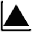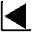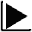Measurement of Friction Coefficient on a Powder Layer and Fluid Dynamic Study## Conclusion

We measured the friction coefficient of a rigid body sliding on a powder layer. This is a model adopted to explain the small apparent friction coefficient exhibited by debris avalanches. In the experiment, flour was mainly used as the powder material and metal disks used as the rigid body that slides over the powder layer. To obtain the friction coefficient, the slide velocity was measured by reading the positions recorded on a digital video camera at 1/60- second intervals.

As a result, we found that friction coefficient became small at a speed higher than a critical value. The obtained friction coefficient was smaller than the normal friction coefficient between rigid bodies. Observation of tracks left on the powder layer indicates that the disk does not press down the powder layer but rather floats on it when the disk slides with small friction.

For comparison, the state of the basal surface of the disk was changed. Small friction coefficients were obtained when using disks with a rough surface onto which particles of powder can stick. Using disks with smooth surfaces gave large friction coefficients and the critical speed of flotation was not found. Additionally,  powder material was changed for comparison. Disks slid in flotation when flour or starch was used, while the critical speed of flotation was not found when silica sand was used.

The dynamic pressure model is proposed as the mechanism providing the force to float a disk. The critical speed derived from this model is consistent with experimental results.

In this experiment, however, we cannot closely observe the state of the powder layer under the disk during the sliding process, and therefore, details of the floating mechanism remain unknown. Also, some experimental results remain unexplained, i.e. the friction coefficient was generally smaller on the starch layer than on the flour layer. Another is that the disk hardly slid on a silica sand layer. Further investigation is needed to explain all these results.

 Measurement of Friction Coefficient on a Powder Layer and Fluid Dynamic Study